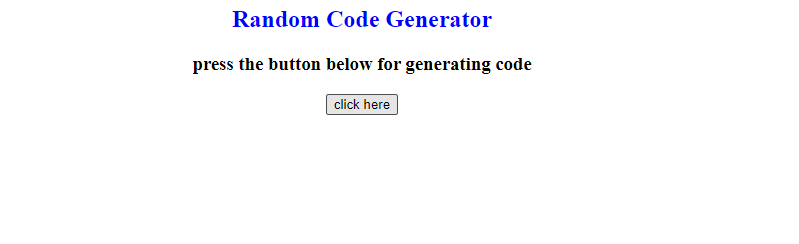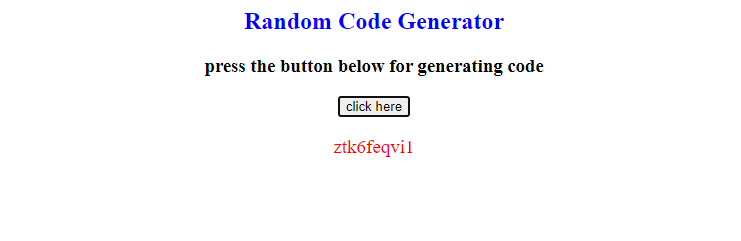# Random Code Generator In Javascript

In this tutorial, we are going to discuss the working of a random code generator and we will also see how to build a random code generator using javascript. But before moving forward let’s have a look at the short introduction to this topic first.

## Introduction

So the question is what is a Random Code Generator? It’s a tool that is used to generate different strings or codes at each time. The random code generator is mostly used in captcha with the web forms.

## Usage of Random Code Generator

On some websites, we have to fill the form first to visit the website, when a user fills the form so at the end of the form there is a captcha that generates a random code, that code is actually a combination of numbers and letters which we have to read and type carefully in the required field, and then we submit the form when the captcha accepts the code. It can be said that the code generator is used for validation in an online registration or contact form.

I hope you got the concept of a random code generator now let’s discuss it works.

## Working of Random Code Generator

This code generator software generates a code of picked characters of the desired length. It selects the random characters or letters and creates a random string of different selected letters according to mentioned length.

## How to create a random code generator using javascript

For building a random code generator software or application we have two ways, First way is by using math.random function and the second way is by using the for loop math.random function.

let’s understand the following examples.

### Example 1

``````<html>

<title>
random code generator using JavaScript

</title>
<body>
<center>
<h2 style="color:blue;">Random Code Generator</h2>

<h3>press the button below for generating code</h3>

<p id="ran_code" style="color: red;

font-size: 19px; font-weight: italic;"> </p>

<script>
var result = document.getElementById('ran_code');
function generate_code() {

result.innerHTML = Math.random().toString(36).slice(2);
}
</script>
</center>

</body>
</html>
``````

#### Code Explanation

In the above code, we wrote the simple HTML structure, in the body tag we first used a center tag and added some headings and designed them by applying CSS, and then we added a button as well with onclick function. We also used a <p> tag with an id named ”ran_code” in which the random code will be printed.

In the script, we declared a variable for storing that element which we will get from the “ran_code” id, and then we created an onclick function named “generate_code”, in the function we used inner HTML with some built-in methods of javascript.

The math.random() method will create random alphanumeric strings or code.

We used the Math.random().toString(36).slice(2) methods, the parameter 36 in tostring() function is the base number that will be converted into strings and integers, mean to say this base number 36 is the combination of 26, alphabets + 0 to 9 numbers for making an alphanumeric string.

We used slice(2) for extracting the text part that will start from position 2.

Basically, the above code will generate the alphanumeric string or a code randomly, the Math.random().toString(36).slice(2) methods will help to build a random code generator.

Let’s Check the results below.

OutputAfter clicking the button:as you can see above our random code generator is working fine. It will display a different random code whenever you refresh the page.

For better understanding let’s move forward to the next example below.

### Example 2

``````<!DOCTYPE html>
<html>

<title></title>
<body>

<script type="text/javascript">
// random code generate program

// declaring all alphabets and numbers
const str_num ='ABCDEFGHIJKLMNOPQRSTUVWXYZabcdefghijklmnopqrstuvwxyz0123456789';

function generate_code(length) {
let output = ' ';
const str_num_Length = str_num.length;
for ( let i = 0; i < length; i++ ) {
output += str_num.charAt(Math.floor(Math.random() * str_num_Length));
}

return output;
}

document.write(generate_code(5));
</script>

</body>
</html>
``````

#### Code Explanation

In the above code, we used a basic HTML structure, and then we wrote the script. In the script, we declared a const “str_num” and stored all the characters of English alphabets, Capital letters, and Small letters and numbers from 0 to9 in it, after that we created a function named “generate_code” and passed length as a parameter.

We set the length of characters with .length and stored it in the “const str_num_Length”.

In the function we used math.random() function that will generate random characters from the declared characters means from (A to Z , a to z , 0 to 9)

And the for loop will print the different generated code on each iteration from the function.

Then we returned the output and printed the code of length 8. Check out the results below.

Output

As you can see in the above output it is displaying a code of length 8, it will display a different random code when you refresh the page.

## Conclusion

In this tutorial we talked about the random code generator, first, we discussed an introduction to Random code Generators then we talked about its usage and their working, we also discussed the making of a random code generator with examples.

We discussed two ways for building a random code generator application and performed some examples using math.random() method and math.random() method with for loop as well.

That’s all for today hope you like the tutorial if you have any queries regarding this article so feel free to contact us below. Thanks for reading.